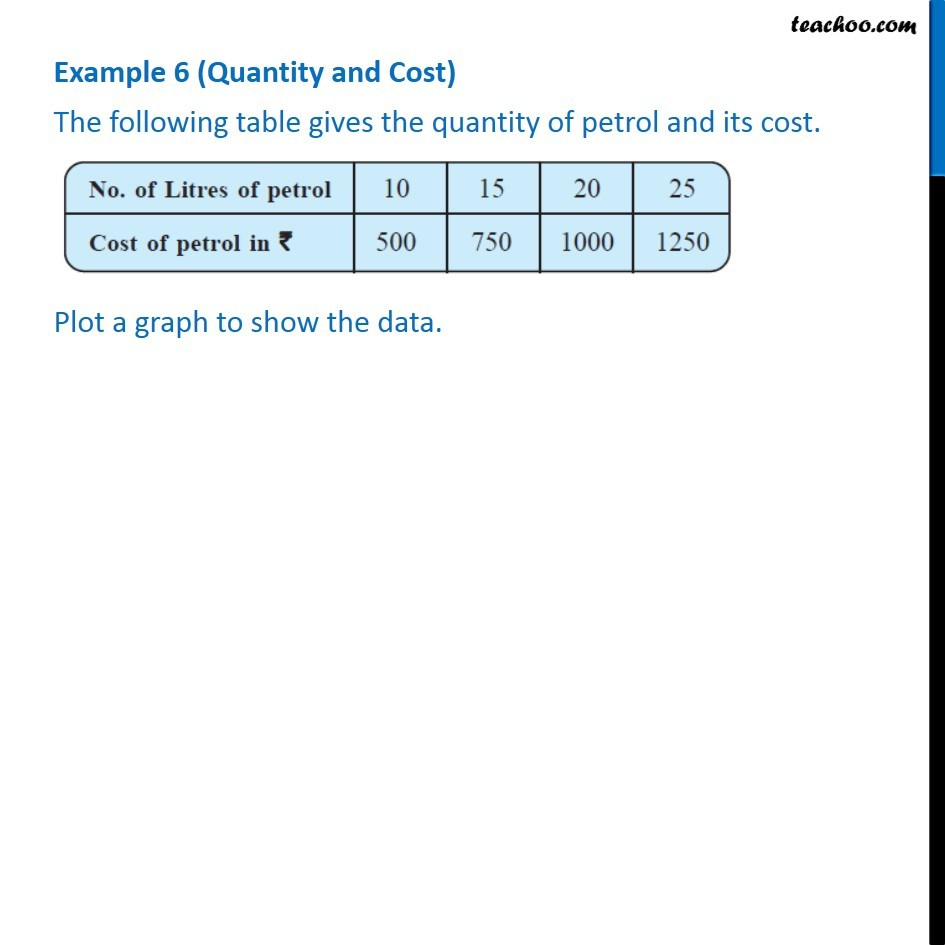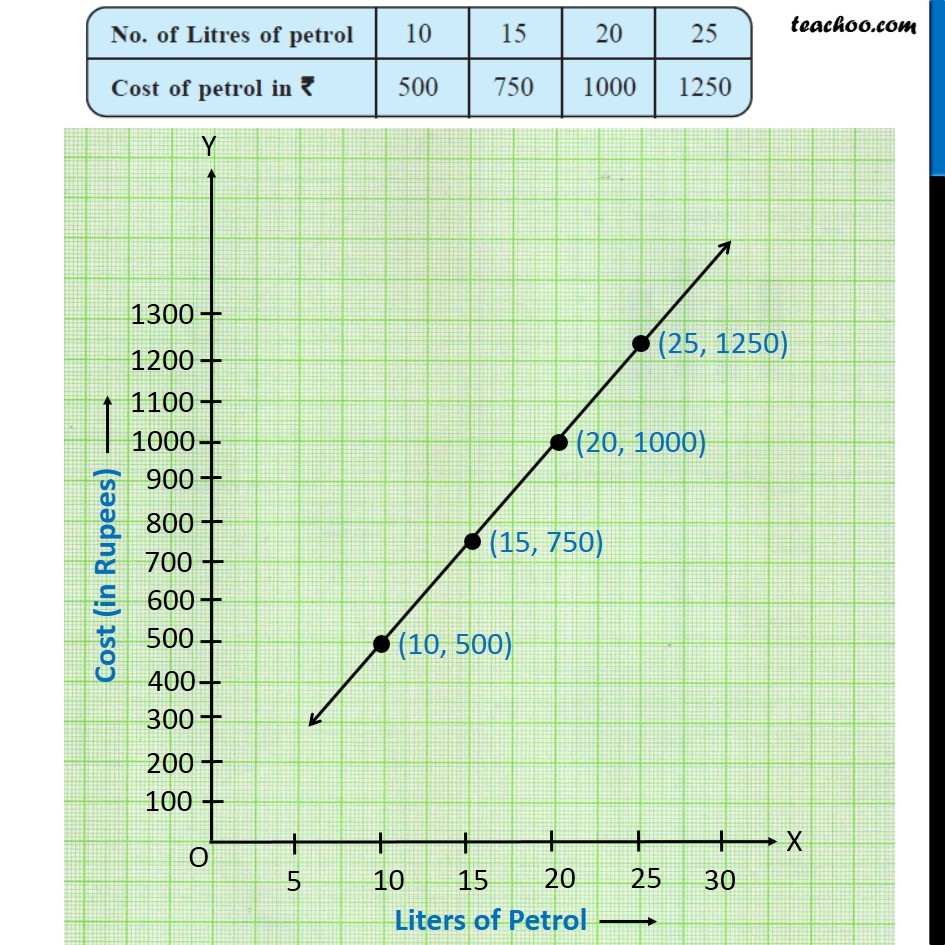Examples

Chapter 13 Class 8 Introduction to Graphs
Serial order wiseLearn in your speed, with individual attention - Teachoo Maths 1-on-1 Class

### Transcript

Example 3 (Quantity and Cost) - Chapter 15 Class 8 Introduction to Graphs The following table gives the quantity of petrol and its cost. No. of liters of petrol | 10 | 15 | 20 | 25 Cost of petrol in rupees | 500 | 750 | 1000 | 1250 Plot a graph to show the data. Plotting graph Putting Cost in rupees in y-axis, from 100 to 1300 and Liters of petrol in x-axis, from 5 to 30 We plot points, and join the line